# 37. Range Object

The Range object, which is the representation of a cell (or cells) on your worksheet, is the most important object of Excel VBA. This chapter gives an overview of the properties and methods of the Range object. Properties are something which an object has (they describe the object), while methods do something (they perform an action with an object).

### Range Examples

Place a command button on your worksheet and add the following code line:

Range(“B3”).Value = 2

Result when you click the command button on the sheet: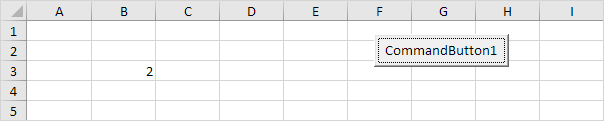Code:

Range(“A1:A4”).Value = 5

Result:Code:

Range(“A1:A2,B3:C4”).Value = 10

Result:Note: to refer to a named range in your Excel VBA code, use a code line like this:

Range(“Prices”).Value = 15

### Cells

Instead of Range, you can also use Cells. Using Cells is particularly useful when you want to loop through ranges.

Code:

Cells(3, 2).Value = 2

Result:Explanation: Excel VBA enters the value 2 into the cell at the intersection of row 3 and column 2.

Code:

Range(Cells(1, 1), Cells(4, 1)).Value = 5

Result: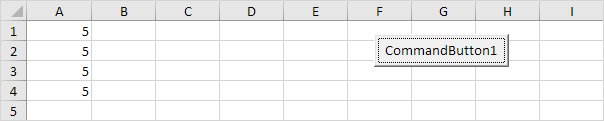### Declare a Range Object

You can declare a Range object by using the keywords Dim and Set.

Code:

Dim example As Range
Set example = Range(“A1:C4”)

example.Value = 8

Result: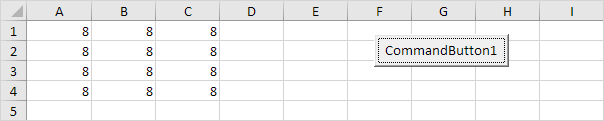### Select

An important method of the Range object is the Select method. The Select method simply selects a range.

Code:

Dim example As Range
Set example = Range(“A1:C4”)

example.Select

Result: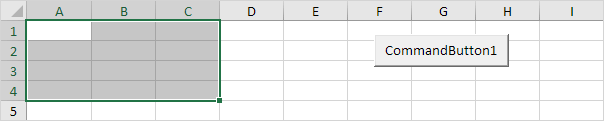Note: to select cells on a different worksheet, you have to activate this sheet first. For example, the following code lines select cell B7 on the third worksheet from the left.

Worksheets(3).Activate
Worksheets(3).Range(“B7”).Select

### Rows

The Rows property gives access to a specific row of a range.

Code:

Dim example As Range
Set example = Range(“A1:C4”)

example.Rows(3).Select

Result:Note: border for illustration only.

### Columns

The Columns property gives access to a specific column of a range.

Code:

Dim example As Range
Set example = Range(“A1:C4”)

example.Columns(2).Select

Result: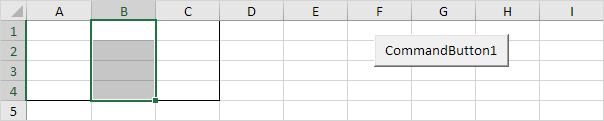Note: border for illustration only.

### Copy/Paste

The Copy and Paste method are used to copy a range and to paste it somewhere else on the worksheet.

Code:

Range(“A1:A2”).Select
Selection.Copy

Range(“C3”).Select
ActiveSheet.Paste

Result: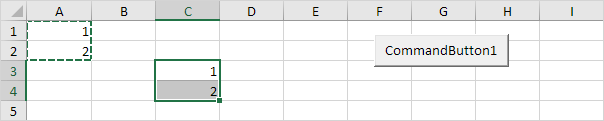Although this is allowed in Excel VBA, it is much better to use the code line below which does exactly the same.

Range(“C3:C4”).Value = Range(“A1:A2”).Value

### Clear

To clear the content of an Excel range, you can use the ClearContents method.

Range(“A1”).ClearContents

or simply use:

Range(“A1”).Value = “”

Note: use the Clear method to clear the content and format of a range. Use the ClearFormats method to clear the format only.

### Count

With the Count property, you can count the number of cells, rows and columns of a range.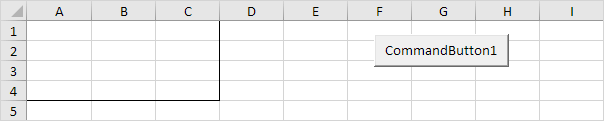Note: border for illustration only.

Code:

Dim example As Range
Set example = Range(“A1:C4”)

MsgBox example.Count

Result: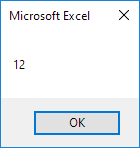Code:

Dim example As Range
Set example = Range(“A1:C4”)

MsgBox example.Rows.Count

Result: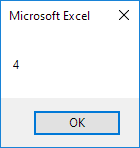Note: in a similar way, you can count the number of columns of a range.